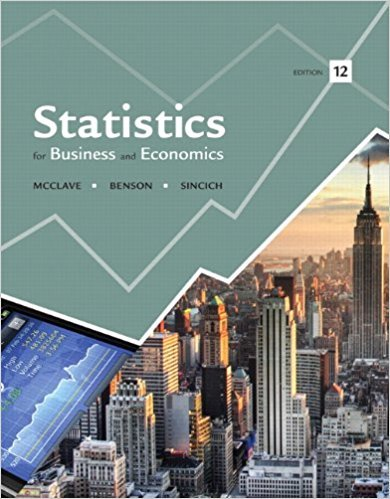×
Get Full Access to Statistics For Business And Economics - 12 Edition - Chapter 11 - Problem 4e
Get Full Access to Statistics For Business And Economics - 12 Edition - Chapter 11 - Problem 4e

×

# Refer to Exercise.The equation (deterministic) for aISBN: 9780321826237 51

## Solution for problem 4E Chapter 11

Statistics for Business and Economics | 12th Edition

• Textbook Solutions
• 2901 Step-by-step solutions solved by professors and subject experts
• Get 24/7 help from StudySoup virtual teaching assistantsStatistics for Business and Economics | 12th Edition

4 5 1 337 Reviews
29
0
Problem 4E

Problem 4E

Refer to Exercise.

The equation (deterministic) for a straight line is

y = β0 + β1x

If the line passes through the point (-2, 4), then x = -2, y = 4 must satisfy the equation; that is,

4= β0 + β1(-2)

Similarly, if the line passes through the point (4, 6), then x = 4, y = 6 must satisfy the equation; that is,

6= β0 + β1x(4)

Use these two equations to solve for β0 and β1 ; then find the equation of the line that passes through the points (-2, 4) and (4, 6).

Find the equations of the lines that pass through the points listed in Exercise.

In each case, graph the line that passes through the given points.

a. (1, 1) and (5, 5)

b. (0, 3) and (3, 0)

c. (-1, 12) and (4, 2)

d. (-6, -32) and (2, 6)

Step-by-Step Solution:
Step 1 of 3

Brand Marketing 9/14/16 2:35 PM September 14, 2016 Brand Value – another value of brand strength – financial value of brand • The premium that accrues to a brand because customers are willing to pay more for the brand than generic products or other competing brands • Brand value = brand equity (correlation) o Even though brand is intangible asset, brand value can be values in dollars. o Intangible asset ▯ Reputation ▯ Patent ▯ Trademarks ▯ Etc. o Easy to value tangible aspects o If you want to know how

Step 2 of 3

Step 3 of 3

##### ISBN: 9780321826237

Unlock Textbook Solution